telescopeѲptics.net          ▪▪▪▪                                             CONTENTS

# 8.2.6. Two-mirror telescopes: miscollimation, close object errors

Miscollimation sensitivity in two-mirror telescopes

Whenever the primary and secondary mirror in a two-mirror system are not optimally positioned, it induces certain amount of axial and off-axis aberrations. In general, induced aberration is proportional to the linear misalignment. While either of the two, or both mirrors can be misaligned, the system sensitivity can be simply shown as misalignment of the secondary relative to the primary. It can be expressed separately for tilt, decenter and despace. By far the dominant aberration resulting from the first two forms of misalignment is axial (independent of the field height) coma. Depending on the sign, it can add up to the "regular" off-axis system coma, or subtract from it, but the most troublesome part is its presence in the field center. As the P-V wavefront error, it can be expressed separately for the tilt as: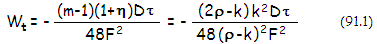and decenter: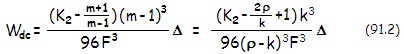τ being the tilt angle in radians, the linear decenter and F the system focal ratio (as before, η is the back focal length in units of primary's focal length and D the aperture diameter). The RMS wavefront error is smaller by a factor 1/321/2. Tilt and decenter are usually both present, so the two errors combine, with the final error given by their sum. Whether they will add or subtract depends on the sign of τ and . Misaligned secondary doesn't induce coma when τ=-∆[1-(m-1)K2/(m+1)]/R2, R2 being the secondary radius of curvature.

Since secondary magnification m=ρ/(ρ-k), relations expressed in terms of secondary magnification m reflect the deviations in either secondary radius of curvature (or, for that matter, primary's radius of curvature, since ρ=R2/R1) or secondary-to-primary separation, defining the minimum relative secondary size k. Thus these relations can also be expressed directly in terms of ρ and k, which can be more convenient for determining the tolerances for a specific system.

Despace (separation) error s in two-mirror systems - positive for larger separation, and vice versa - results in change in the relative height of the marginal ray at the secondary k into k'=k+(s/ƒ1), and secondary magnification m into m'=ρ/(ρ-k'). The most significant aberration it induces is lower-order spherical. Resulting wavefront error is obtained by substituting k' and m' for k and m in Eq. 81 (top), or simply k' for k (bottom). The P-V wavefront error at the best focus can be also expressed in terms of secondary magnification alone as: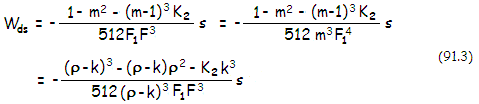with F1 being the primary's focal ratio. For given nominal value of s the wavefront error is independent of the aperture; for relative s value (in units of the primary's focal length), it changes in proportion to it.

Despace also induces coma, insignificant in comparison. According to Schroeder's relation for angular transverse coma created by despace (p114), its ratio to the coma of classical Cassegrain (or paraboloid of identical F-number) is given by -[(2m2-1)(m-β)+(2m(m+1)]ms/(1+β). The minus sign indicates that this coma adds to the system coma for negative, and subtracts for positive values of s; in aplanatic two-mirror systems it represents the amount of system coma.

Eq. 91.3 shows that Dall-Kirkham with K2=0 has significantly lower sensitivity to despace compared to both, classical and aplanatic Cassegrain (Ritchey-Chrétien), with the latter being the most sensitive. However, despace sensitivity is lowest in the Gregorian arrangement, for which the sum in the brackets is smaller than in a comparable Dall-Kirkham, due to numerically low negative secondary conic of the former.

Note that the separation change s is negative when the mirror separation decreases, resulting in larger minimum relative secondary size k' (primary's f.l. is numerically negative, according to the sign convention). From Eq. 91.4 it is easy to see that larger than optimum mirror separation (i.e. positive s) induces under-correction (positive in sign wavefront error at the best focus), and that smaller mirror separation (negative s) induces over-correction.

Plots below shows the P-V wavefront error (λ=550nm) due to miscollimation for selected two mirror systems: ƒ/3.33/10 and ƒ/4/16 each, classical Cassegrain (Cass) and Dall-Kirkham (DK), as well as for ƒ/2.5/7.5 and ƒ/3.33/10 Ritchey-Chrétien (RC). The ƒ/10 systems are for direct comparison. Tilt-induced coma (left) is independent of the secondary conic, hence it is identical for any two-mirror arrangement with given secondary magnification (m), aperure (D, 200mm here), and back focal length (η, 0.2 for all systems). At ƒ/10, both decenter and despace sensitivity are significantly lower in the DK than Cassegrain and RC, while RC is moderately more sensitive than Cassegrain. At the typical focal ratio, however, (~ƒ/8 RC and ~ƒ/15 Cassegrain) Cassegrain is significantly less sensitive.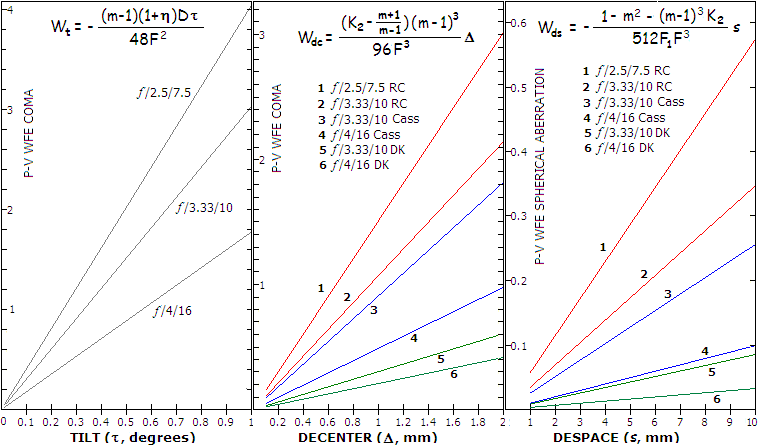For relatively close objects, when magnification of the primary - given by m1=-ƒ1/(o-ƒ1), ƒ1 being the primary focal length and o the object distance - is appreciably greater than zero, Eq. 9 applies to both mirrors. The aberration contribution of the primary changes from (K1+1) into [K1+(1-2ψ)2], with ψ=ƒ1/o being the primary focal length in units of the object distance. Also, due to the extended converging cone of the primary, both, relative height of the marginal ray at the secondary k and secondary magnification m increase. The height k becomes k'=(1-ψ)k+ψ, and secondary magnification m becomes m'=ρ'/(ρ'-k'), with ρ'=(1-ψ)ρ. For the secondary, the effective primary focal ratio is now F1/(1-ψ). With these changes, after substituting k', ρ' and m' for k, ρ and m in Eq. 81 (bottom) the system P-V wavefront error of spherical aberration at the best focus becomes: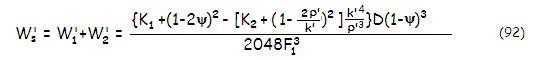Since the secondary conic K2 is a factor for the aberration contribution of the secondary, sensitivity of two-mirror telescopes to reduction in object distance varies with the system type. Plots below, based on Eq. 92, show spherical aberration induced by close object observing.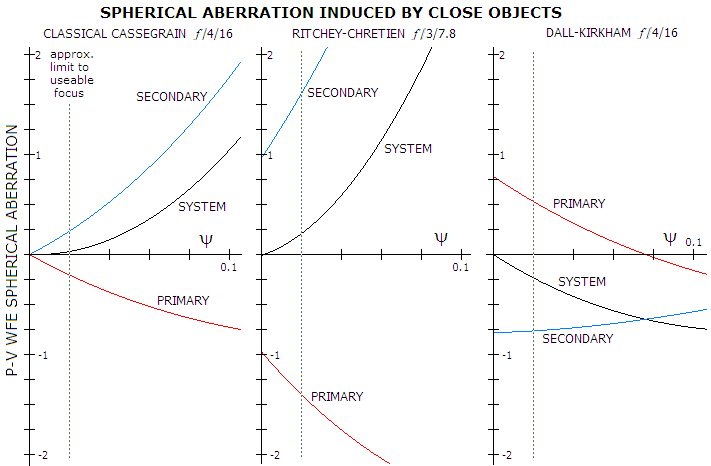With classical Cassegrain (left), both primary and secondary induce zero spherical aberration with object at infinity. As object distance diminishes, paraboloidal primary induced overcorrection, but the hyperboloidal secondary induces even more undercorrection, at a higher rate, resulting in the increase of system undercorrection. The two hyperboloidal mirrors of the Ritchey-Chretien (RC), figured to correct coma, each induces spherical aberration that exactly offsets for object at infinity. For closer objects, primary's overcorrection increases more slowly than secondary's undercorrection, resulting in system undercorrection. RC error is significantly larger than for the Cassegrain, mainly due to the faster primary.

Similarly, undercorrection of Dall-Kirkham (DK) ellipsoidal primary is offset by overcorrection of the secondary for object at infinity. For closer objects, aberration contribution by both mirrors diminishes, but more slowly for the secondary, with the system becoming increasingly overcorrected. Error induced to the DK is significantly greater than that in the Cassegrain, approaching 1/4 wave at the limit of useable focus range.

8.2.5. Loveday telescope   ▐    8.3. Three-mirror telescopes